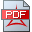www.riemann-hypothesis.de
www.goldbach-conjecture.de

A Kummer function based zeta function theory to solve the Riemann Hypothesis and a proposed two semi-circle method to solve the Goldbach conjecture.

The Riemann Hypothesis

The Riemann Hypothesis states that all non-trivial zeros of the zeta function have real-part one-half.  The conceptual linkage to the UFT pages is given by the Hilbert-Polya conjecture and the Berry-Keating conjecture.

Alternatively to the current Gaussian function based zeta function theory a Kummer function based zeta function theory is proposed to enable the verification of several RH criteria.

The Goldbach conjecture

The binary Goldbach conjecture states that every positive even number n > 2 is the sum of two primes.

The Hardy-Littlewood circle method is based on the set of integers, which are the zeros of the orthonormal (Fourier series based) basis functions of the L(2) Hilbert space on the unit circle. All attempts applying the Hardy-Littlewood circle method to prove the binary Goldbach conjecture failed due to the insufficient (purely Weyl sum based) bounds for the minor arcs.

An alternative two semi-circle method is proposed, which is built on non-harmonic Fourier series and related Riesz bases on the unit circle. They are built on the imaginary parts a(n) of the zeros of the considered Kummer function and the real, negative zeros of the Digamma function. Both sequences enjoy similar properties enabling N. Levinson's "gap and density theorems". The theory of non-harmonic Fourier series is enabled by appropriately defined „retarded / condensed“ sequences in the form b(n):=(3*a(n)+a(n+1))/4 fulfilling the Kadec condition. Those sequences are supposed to govern the "even-semi-circle" resp. the "odd-semi-circle". At the same time, both semi-circles can be mapped onto the negative real line until +1/2 (hosting all negative zeros of the Digamma function) and the positive critical line (not hosting any zero of the considered Kummer function).Braun K., A toolbox to solve the RH and to build a non-harmonic Fourier series based two-semicircle methodBraun, K., The Kummer conjecture and the two-semicircle method

Earlier supporting papersBraun K., A Kummer function based Zeta function theory to prove the Riemann Hypothesis and the binary Goldbach conjecture, July 31, 2019Braun K., A Kummer function based Zeta function theory to prove the Riemann Hypothesis and the Goldbach conjecture

A great detailed analysis of Riemann's article entitled, "On the Numbers of Primes Less Than a Given Magnitude" is provided inDittrich W., On Riemann s Paper, On the Number of Primes Less Than a Given Magnitude